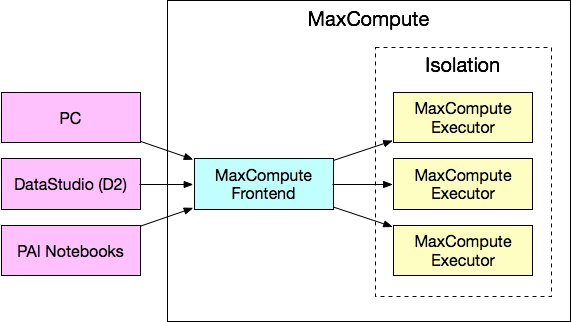PyODPS 是 MaxCompute(ODPS) Python SDK，为 MaxCompute 对象提供了 Python 端的操作接口，同时，对于熟悉 Pandas 的用户来说，它提供了 DataFrame API 来用类似 Pandas 的接口进行大规模数据分析以及处理，并能够方便的将 MaxCompute 的分布式 DataFrame 向本地 Pandas DataFrame 转换。正因为如此的方便灵活，在实际使用过程中，不少用户容易把 PyODPS 用成单机处理模式，没有最大限度发挥 MaxCompute 分布式运算的性能。本文主要介绍如何高效地使用 PyODPS 处理数据。

## 概念## 一个例子：分词

``````import jieba
t = o.get_table('word_split')
out = []
words = list(jieba.cut(r))
#
# 处理逻辑，产生出 processed_data
#
out.append(processed_data)
out_t = o.get_table('words')
with out_t.open_writer() as writer:
writer.write(out)``````

## 高效的分词

``````from odps import output
out_table = o.get_table('words')
df = o.get_table('word_split').to_df()
@output(out_table.schema.names, out_table.schema.types)
def handle(row):
import jieba
words = list(jieba.cut(r))
#
# 处理逻辑，产生出 processed_data
#
yield processed_data
df.apply(handle, axis=1).persist(out_table)``````

## One Comment

•### 留言

博主你好，看了你的datawork处理分词，我想请问一下，pyodps教程使用archive，zip资源用的是 iris.chinese.map(test, resources=[file_object]).execute(hints=hints, libraries=libraries) 请问有别的方法可以实现test方法引用jieba吗